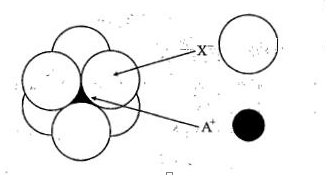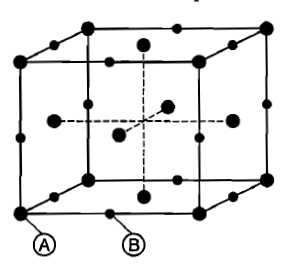# NEET Questions Solved

Total volume of atoms present in a face centred cubic unit cell of a metal is ( r is atomic radius):

(A) 20/3 $\pi$r3

(B)  24/3 $\pi$r3

(C)  12/3 $\pi$r3

(D)  16/3 $\pi$r3

Concept Videos :-

#5 | Simple Cubic Unit Cell: Coordination Number
#10 | FCC Unit Cell: Coordination Number
#15 | Tetrahedral Voids
#16 | Octahedral Voids
#17 | Position of Tetrahedral Voids in FCC
#18 | Position of Octahedral Voids in FCC
#19 | Hexagonal Primitive (HP) Unit Cell
#22 | Coordination Number of HP Unit Cel

Concept Questions :-

Complete Question Bank + Test Series
Complete Question Bank

Difficulty Level:

The arrangement of Xions around A+ ion in solid AX is given in the figure (not drawn to scale).
If the radius of X is 250 pm,the radius of A+ is:(a)104 pm
(b)125 pm
(c)183 pm
(d) 57 pm

Concept Videos :-

#5 | Simple Cubic Unit Cell: Coordination Number
#10 | FCC Unit Cell: Coordination Number
#15 | Tetrahedral Voids
#16 | Octahedral Voids
#17 | Position of Tetrahedral Voids in FCC
#18 | Position of Octahedral Voids in FCC
#19 | Hexagonal Primitive (HP) Unit Cell
#22 | Coordination Number of HP Unit Cel

Concept Questions :-

Complete Question Bank + Test Series
Complete Question Bank

Difficulty Level:

Select the correct statement (s)-

(a) The C.N. of cation occupying a tetrahedral hole is 4.

(b) The C.N. of cation occupying a octahedral hole is 6.

(c) In schottky defects, density of the lattice decreases,

(A) a,b

(B) b,c

(C) a,b,c

(D) a,c

Concept Videos :-

#5 | Simple Cubic Unit Cell: Coordination Number
#10 | FCC Unit Cell: Coordination Number
#15 | Tetrahedral Voids
#16 | Octahedral Voids
#17 | Position of Tetrahedral Voids in FCC
#18 | Position of Octahedral Voids in FCC
#19 | Hexagonal Primitive (HP) Unit Cell
#22 | Coordination Number of HP Unit Cel

Concept Questions :-

Complete Question Bank + Test Series
Complete Question Bank

Difficulty Level:

Among the following types of voids. which one is the largest void:-

(A) Triangular system
(B) Tetragonal system
(C) Monoclinic system
(D) Octahedral

Concept Videos :-

#5 | Simple Cubic Unit Cell: Coordination Number
#10 | FCC Unit Cell: Coordination Number
#15 | Tetrahedral Voids
#16 | Octahedral Voids
#17 | Position of Tetrahedral Voids in FCC
#18 | Position of Octahedral Voids in FCC
#19 | Hexagonal Primitive (HP) Unit Cell
#22 | Coordination Number of HP Unit Cel

Concept Questions :-

Complete Question Bank + Test Series
Complete Question Bank

Difficulty Level:

The arrangement ABC ABC.. is referred to as.

(a) octahedral close packing

(b) hexagonal clase packing

(c) tetrahedral close packing

(d) cubic close packing

Concept Videos :-

#5 | Simple Cubic Unit Cell: Coordination Number
#10 | FCC Unit Cell: Coordination Number
#15 | Tetrahedral Voids
#16 | Octahedral Voids
#17 | Position of Tetrahedral Voids in FCC
#18 | Position of Octahedral Voids in FCC
#19 | Hexagonal Primitive (HP) Unit Cell
#22 | Coordination Number of HP Unit Cel

Concept Questions :-

Complete Question Bank + Test Series
Complete Question Bank

Difficulty Level:

The ratio of cations to anion in a closed pack tetrahedral is:

(a) 0.414
(b) 0.225
(c) 0.02
(d) none of these

Concept Videos :-

#5 | Simple Cubic Unit Cell: Coordination Number
#10 | FCC Unit Cell: Coordination Number
#15 | Tetrahedral Voids
#16 | Octahedral Voids
#17 | Position of Tetrahedral Voids in FCC
#18 | Position of Octahedral Voids in FCC
#19 | Hexagonal Primitive (HP) Unit Cell
#22 | Coordination Number of HP Unit Cel

Concept Questions :-

Complete Question Bank + Test Series
Complete Question Bank

Difficulty Level:

For a solid with the following structure, the co-ordination number of the point B is:(a) 3

(b) 4

(c) 5

(d)6

Concept Videos :-

#5 | Simple Cubic Unit Cell: Coordination Number
#10 | FCC Unit Cell: Coordination Number
#15 | Tetrahedral Voids
#16 | Octahedral Voids
#17 | Position of Tetrahedral Voids in FCC
#18 | Position of Octahedral Voids in FCC
#19 | Hexagonal Primitive (HP) Unit Cell
#22 | Coordination Number of HP Unit Cel

Concept Questions :-

Complete Question Bank + Test Series
Complete Question Bank

Difficulty Level:

A crystal formula AB3 has A ions at the cube corners and B ions at the edge centres. The coordination number of A and B respectively

(A) 6 and 6

(B) 2 and 6

(C) 6 and 2

(D) 8 and 8

Concept Videos :-

#5 | Simple Cubic Unit Cell: Coordination Number
#10 | FCC Unit Cell: Coordination Number
#15 | Tetrahedral Voids
#16 | Octahedral Voids
#17 | Position of Tetrahedral Voids in FCC
#18 | Position of Octahedral Voids in FCC
#19 | Hexagonal Primitive (HP) Unit Cell
#22 | Coordination Number of HP Unit Cel

Concept Questions :-

Complete Question Bank + Test Series
Complete Question Bank

Difficulty Level:

Compute the percentage void space per unit volume of unit cell in zinc fluoride structure.

1. 15.03%

2. 22.18%

3. 18.23%

4. 25.07%

Concept Videos :-

#5 | Simple Cubic Unit Cell: Coordination Number
#10 | FCC Unit Cell: Coordination Number
#15 | Tetrahedral Voids
#16 | Octahedral Voids
#17 | Position of Tetrahedral Voids in FCC
#18 | Position of Octahedral Voids in FCC
#19 | Hexagonal Primitive (HP) Unit Cell
#22 | Coordination Number of HP Unit Cel

Concept Questions :-

Complete Question Bank + Test Series
Complete Question Bank

Difficulty Level:

8 : 8 co-ordination of CsCl is found to change into 6 : 6
co-ordination on:

(a) applying pressure
(b) increasing temperature
(c) both (a) and (b)
(d) none of these

Concept Videos :-

#5 | Simple Cubic Unit Cell: Coordination Number
#10 | FCC Unit Cell: Coordination Number
#15 | Tetrahedral Voids
#16 | Octahedral Voids
#17 | Position of Tetrahedral Voids in FCC
#18 | Position of Octahedral Voids in FCC
#19 | Hexagonal Primitive (HP) Unit Cell
#22 | Coordination Number of HP Unit Cel